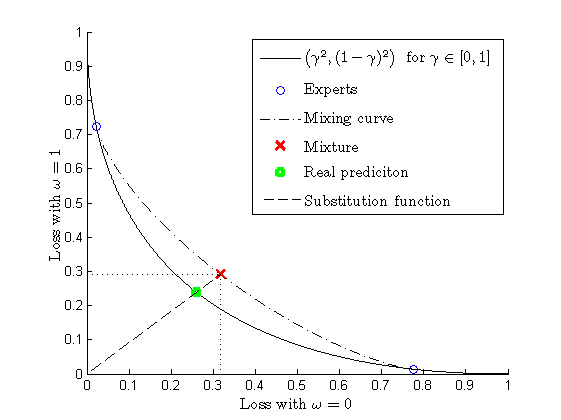# Strong Aggregating Algorithm

Strong Aggregating Algorithm is one of the exponential weights algorithms. It usually enjoys the strongest theoretical performance guarantees, although its computational efficiency may not be as good as some of its competitors'. In the case of a finite number of experts {$K$}, it makes predictions according to the following scheme (some explanations can be found after the description of the algorithm):

Initialize weights of experts {$w_k:=1/K$}, {$k=1,\ldots,K$}.
Initialize cumulative losses {$L_t:=0, L_t^k:=0, k=1,\ldots,K$}.
FOR {$t=1,2,\dots$}
Experts announce predictions {$\gamma_t^k \in \Gamma, k=1,\ldots,K$}.
Calculate the generalized prediction {$g_t(\omega)=-\frac{1}{\eta}\ln\sum_{k=1}^K w_k e^{-\eta \lambda(\omega,\gamma_t^k)}, \forall \omega \in \Omega$}.
Algorithm announces {$\gamma_t := S(g_t) \in \Gamma$}, where {$S$} is the chosen substitution function.
Reality announces {$\omega_t\in\Omega$}.
{$L_t:=L_{t-1}+\lambda(\omega_t,\gamma_t)$}.
{$L_t^k:=L_{t-1}^k+\lambda(\omega_t,\gamma_t^k), k=1,\ldots,K$}.
Update the weights {$w_k := w_k e^{-\eta \lambda(\omega_t,\gamma_t^k)}$} and normalize them {$w_k := w_k / \sum_{k=1}^K w_k$}, {$k=1,\ldots,K$}.
END FOR.Figure 1. Aggregating algorithm in case of two possible outcomes,
two experts, and square-loss function
.

In this description, {$\lambda(\omega,\gamma): \Omega \times \Gamma \to \mathbb{R}$} is the loss function chosen for the current game, and {$\eta$} is the learning rate, a parameter of the algorithm. The protocol above means that Strong Aggregating Algorithm takes the losses suffered by the experts, transfers them into an exponential space by {$e^{-\eta \lambda(\omega,\gamma^k)}$}, mixes them using the weights of experts, and transfers them back from the exponential space; this way we receive the so-called generalized prediction. It is clear that the generalized prediction is not always a real prediction, and one should apply the substitution function to it. The substitution function {$S$} is such a function that for {$\gamma=S(g)$} we have {$\lambda(\omega,\gamma) \le c(\eta) g(\omega)$} for the smallest possible {$c(\eta)\ge1$} and for all {$\omega\in\Omega$}. For various loss functions it can be shown that

{$L_T \le c(\eta)L_T^k + a(\eta)\ln K$}

for all {$T$} and {$k$}, where {$L_T$} is the loss suffered by the algorithm over the first {$T$} trials and {$L_T(k)$} is the loss suffered by the {$k$}th expert over the first {$T$} trials. For the mixable loss functions {$c(\eta)=1, a(\eta)=1/ \eta$}. In particular, {$c(\eta)=1$} and {$a(\eta)=1/2$} for the square-loss and the case when {$\Omega=[0,1], \Gamma=[0,1]$}.

For the online linear regression problem with bounded signal {$x: ||x_t||_\infty \le B$}, Vovk (2001) proves that for the square-loss function Aggregating Algorithm (Aggregating Algorithm Regression) can achieve

{$L_T \le \inf_\theta (L_T(\theta)+a||\theta||^2_2) + nY^2\ln(TB^2/a+1)$},

where {$L_T(\theta)$} is the loss of any linear function of {$x$}.

Important special cases:

### Bibliography

• Vladimir Vovk. Aggregating strategies. In: Proceedings of the 3rd Annual Workshop on Computational Learning Theory (ed by M Fulk and J Case), pp 371 - 383. San Mateo, CA: Morgan Kaufmann, 1990.
• Vladimir Vovk. A game of prediction with expert advice. Journal of Computer and System Sciences 56:153 - 173, 1998.
• Vladimir Vovk. Competitive on-line statistics. International Statistical Review 69, 213–248 (2001).